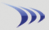Subscription & data-feed pricing Class schedule

New account application Trading resources
Margin rates Stock & option commissions

Attention: Discussion forums are read-only for extended maintenance until further notice.
 Welcome Guest, please sign in to participate in a discussion. Search | Active Topics |

 Cup & Handle PCF Topic Rating:Previous Topic · Next Topic Watch this topic · Print this topic ·
tsisung
 Posted : Thursday, November 05, 2009 12:14:25 AM
Registered User
Joined: 4/3/2005
Posts: 41
This is what I developed and use.  Thoughts/feedback appreciated.  Tim

(MAXH10 < MAXH101.29 AND C <= MAXH129.1 AND C1 <= MAXH128.2 AND C2 <= MAXH127.3 AND C3 <= MAXH126.4 AND C4 <= MAXH125.5 AND C5 <= MAXH124.6 AND C6 <= MAXH123.7 AND C7 <= MAXH122.8 AND C8 <= MAXH121.9 AND C9 <= MAXH120.10 AND C10 <= MAXH119.11 AND C11 <= MAXH118.12 AND C12 <= MAXH117.13 AND C13 <= MAXH116.14 AND C14 <= MAXH115.15 AND C15 <= MAXH114.16 AND C16 <= MAXH113.17 AND C17 <= MAXH112.18 AND C18 <= MAXH111.19 AND C19 <= MAXH110.20 AND C20 <= MAXH109.21 AND C21 <= MAXH108.22 AND C22 <= MAXH107.23 AND C23 <= MAXH106.24 AND C24 <= MAXH105.25 AND C25 <= MAXH104.26 AND C26 <= MAXH103.27 AND C27 <= MAXH102.28 AND C28 <= MAXH101.29 AND C29 <= MAXH100.30 AND C >= AVGC50 AND C / MAXH130 >= .80 AND (MINC65 - MAXC130) / MAXC130 >= ( - .55) AND (MINC65 - MAXC130) / MAXC130 <= ( - .12) AND MAXC10 / MINC10 <= 1.25 AND (C - MINC65) / (MAXC130 - MINC65) > .5)
 Posted : Friday, January 08, 2010 8:33:27 PM
Registered User
Joined: 8/4/2009
Posts: 4
Nice results for that scan. Thanks for sharing it?
bud11
 Posted : Wednesday, January 20, 2010 4:50:28 PM
Registered User
Joined: 12/22/2009
Posts: 10
can I paste that in if I have the gold version???
Bruce_L
 Posted : Wednesday, January 20, 2010 4:56:07 PMWorden Trainer

Joined: 10/7/2004
Posts: 65,138
bud11,
It appears to be a standard Personal Criteria Formula that should work in all versions of TeleChart.

How to create a Personal Criteria Forumula (PCF)

-Bruce
Personal Criteria Formulas
TC2000 Support Articles
benpar
 Posted : Friday, April 13, 2012 3:52:28 PM
Registered User
Joined: 6/28/2005
Posts: 9

I would like to scan stocks using a 5-day RSI with a  reading of 10 or less plus a volume of at least 1M shares a day. I am using TC2000 v 12. Any suggestions? Thanks.

Bruce_L
 Posted : Friday, April 13, 2012 3:56:10 PMWorden Trainer

Joined: 10/7/2004
Posts: 65,138

If it is the plain RSI indicator, a Condition Formula for this could be written as:

RSI 5 <= 10 AND V >= 1000000

If it is the RSI - Wilder's indicator, a Condition Formula for this could be written as:

50 * (C - XAVGC9.1) / 5 / (.20001701556587 * (ABS(C - C1) + .8 * (ABS(C1 - C2) + .8 * (ABS(C2 - C3) + .8 * (ABS(C3 - C4) + .8 * (ABS(C4 - C5) + .8 * (ABS(C5 - C6) + .8 * (ABS(C6 - C7) + .8 * (ABS(C7 - C8) + .8 * (ABS(C8 - C9) + .8 * (ABS(C9 - C10) + .8 * (ABS(C10 - C11) + .8 * (ABS(C11 - C12) + .8 * (ABS(C12 - C13) + .8 * (ABS(C13 - C14) + .8 * (ABS(C14 - C15) + .8 * (ABS(C15 - C16) + .8 * (ABS(C16 - C17) + .8 * (ABS(C17 - C18) + .8 * (ABS(C18 - C19) + .8 * (ABS(C19 - C20) + .8 * (ABS(C20 - C21) + .8 * (ABS(C21 - C22) + .8 * (ABS(C22 - C23) + .8 * (ABS(C23 - C24) + .8 * (ABS(C24 - C25) + .8 * (ABS(C25 - C26) + .8 * (ABS(C26 - C27) + .8 * (ABS(C27 - C28) + .8 * (ABS(C28 - C29) + .8 * (ABS(C29 - C30) + .8 * (ABS(C30 - C31) + .8 * (ABS(C31 - C32) + .8 * (ABS(C32 - C33) + .8 * (ABS(C33 - C34) + .8 * (ABS(C34 - C35) + .8 * (ABS(C35 - C36) + .8 * (ABS(C36 - C37) + .8 * (ABS(C37 - C38) + .8 * (ABS(C38 - C39) + .8 * (ABS(C39 - C40) + .8 * (ABS(C40 - C41) + .8 * (ABS(C41 - C42)))))))))))))))))))))))))))))))))))))))))))) + 50 < 10 AND V >= 1000000

-Bruce
Personal Criteria Formulas
TC2000 Support Articles
rdhawan
 Posted : Sunday, December 29, 2013 3:36:10 PM
Registered User
Joined: 4/18/2011
Posts: 2

QUOTE (Bruce_L)
bud11,
It appears to be a standard Personal Criteria Formula that should work in all versions of TeleChart.

How to create a Personal Criteria Forumula (PCF)

I am looking condition to add "Shares Ourstanding" i can not find it, please help

Bruce_L
 Posted : Monday, January 06, 2014 10:22:10 AMWorden Trainer

Joined: 10/7/2004
Posts: 65,138

Outstanding Shares is not one of the fundamental system criteria available in TC2000.

-Bruce
Personal Criteria Formulas
TC2000 Support Articles
Users browsing this topic
Guest-1

 Forum Jump Customer Training & Support - Ask a Trainer - TC2000 version 12/18 - Ask a Trainer - TC2000 version 7 - Ask a Trainer - StockFinder 5.0 - PCFs, EasyScan and Custom Indicators General Discussions - Stock and Market Talk - TC2000 version 12 or 18 - TC2000 version 7 - StockFinder 5.0 - RealCode for StockFinder 5.0 Tutorial Videos - TC2000 version 12 tutorial videos - TC2000 version 7 tutorial videos You cannot post new topics in this forum. You cannot reply to topics in this forum. You cannot delete your posts in this forum. You cannot edit your posts in this forum. You cannot create polls in this forum. You cannot vote in polls in this forum.